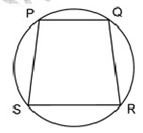In the figure above, PQRS is a cyclic quadrilateral, where PQ = p cm, QR = q cm, RS = r cm and PS = s cm. If (PQ) (QR) = 3(PS) (RS) and ∠PQR =1200, then s =